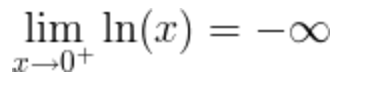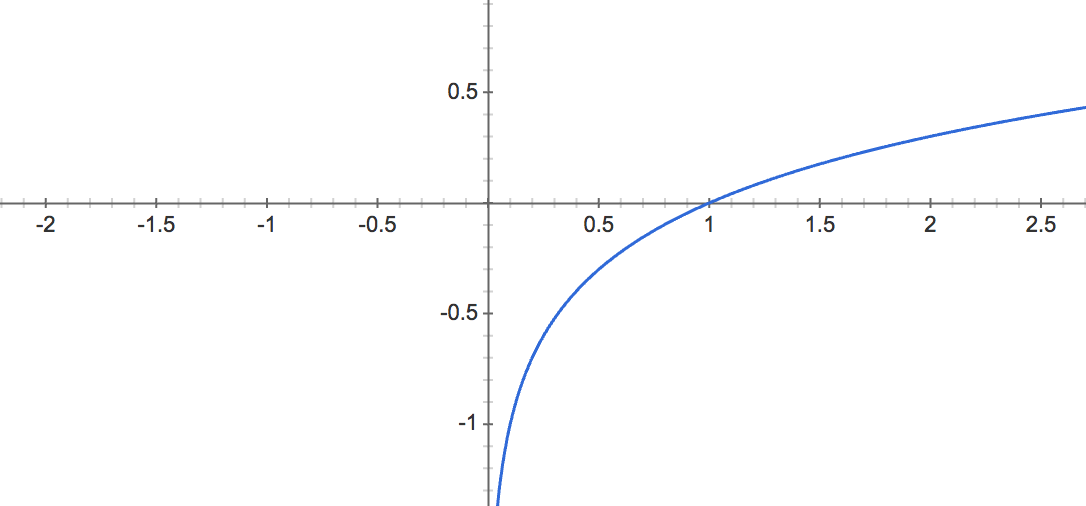# Natural Logarithm – ln(x)

Natural logarithm is the logarithm to the base e of a number.

Contents

### Definition of natural logarithm

When

e y = x

Then base e logarithm of x is

ln(x) = loge(x) = y

The e constant or Euler’s number is:

e ≈ 2.71828183

### Ln as inverse function of exponential function

The natural logarithm function ln(x) is the inverse function of the exponential function ex.

For x>0,

f (f -1(x)) = eln(x) = x

Or

-1(f (x)) = ln(ex) = x

### Natural logarithm rules and properties

Logarithm product rule

The logarithm of the multiplication of x and y is the sum of logarithm of x and logarithm of y.

logb(x ∙ y) = logb(x) + logb(y)

For example:

log8(4 ∙ 5) = log8(4) + log8(5)

Logarithm quotient rule

The logarithm of the division of x and y is the difference of logarithm of x and logarithm of y.

logb(x / y) = logb(x) – logb(y)

For example:

log8(4 / 5) = log8(4) – log8(5)

Logarithm power rule

The logarithm of x raised to the power of y is y times the logarithm of x.

logb(x y)y ∙ logb(x)

For example:

log8(39) = 9 ∙ log8(3)

Derivative of natural logarithm

The derivative of the natural logarithm function is the reciprocal function.

When

f (x) = ln(x)

The derivative of f(x) is:

f ‘ (x) = 1 / x

Integral of natural logarithm

The integral of the natural logarithm function is given by:

When

f (x) = ln(x)

The integral of f(x) is:

∫ f (x)dx = ∫ ln(x)dx = x ∙ (ln(x) – 1) + C

Ln of 0

The natural logarithm of zero is undefined:

ln(0) is undefined

The limit near 0 of the natural logarithm of x, when x approaches zero, is minus infinity:Ln of 1

The natural logarithm of one is zero:

ln(1) = 0

Ln of infinity

The limit of natural logarithm of infinity, when x approaches infinity is equal to infinity:

lim ln(x) = ∞, when x→∞

### Complex logarithm

For complex number z:

z = re = x + iy

The complex logarithm will be (n = …-2,-1,0,1,2,…):

log z = ln(r) + i(θ+2nπ) = ln(√(x2+y2)) + i·arctan(y/x))

### Graph of ln(x)

ln(x) is not defined for real non positive values of x: